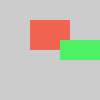# np_pixels[]#

The `np_pixels[]` array contains the values for all the pixels in the display window.

## Examples#```def setup():
pink = [255, 255, 102, 204]
py5.np_pixels[:py5.height//2, :, :] = pink
py5.update_np_pixels()
``````def setup():
py5.np_pixels[20:50, 30:70, 1:] = [240, 100, 80]
py5.np_pixels[40:60, 60:, 1:] = [80, 240, 100]
py5.update_np_pixels()
```

## Description#

The `np_pixels[]` array contains the values for all the pixels in the display window. Unlike the one dimensional array pixels[], the `np_pixels[]` array organizes the color data in a 3 dimensional numpy array. The size of the array’s dimensions are defined by the size of the display window. The first dimension is the height, the second is the width, and the third represents the color channels. The color channels are ordered alpha, red, green, blue (ARGB). Every value in `np_pixels[]` is an integer between 0 and 255.

This numpy array is very similar to the image arrays used by other popular Python image libraries, but note that many of them will arrange the channels in a different order such as RGBA or BGR.

When the pixel density is set to higher than 1 with the pixel_density() function, the size of `np_pixels[]`’s height and width dimensions will change. See the reference for pixel_width or pixel_height for more information. Nothing about `np_pixels[]` will change as a result of calls to color_mode().

Much like the pixels[] array, there are load and update methods that must be called before and after making changes to the data in `np_pixels[]`. Before accessing `np_pixels[]`, the data must be loaded with the load_np_pixels() method. If this is not done, `np_pixels` will be equal to `None` and your code will likely result in Python exceptions. After `np_pixels[]` has been modified, the update_np_pixels() method must be called to update the content of the display window.

To set the entire contents of `np_pixels[]` to the contents of another properly sized numpy array, consider using set_np_pixels().

Updated on September 01, 2022 16:36:02pm UTC